# AP Calculus BC : Radius and Interval of Convergence of Power Series

## Example Questions

### Example Question #58 : Ap Calculus Bc

Which of following intervals of convergence cannot exist?For any, the interval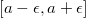for some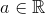For any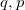such that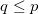, the interval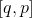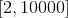Explanation: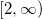cannot be an interval of convergence because a theorem states that a radius has to be either nonzero and finite, or infinite (which would imply that it has interval of convergence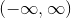). Thus,can never be an interval of convergence.

### Example Question #3003 : Calculus Ii

Find the interval of convergence offor the series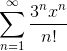.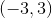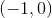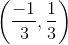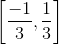Explanation:

Using the root test,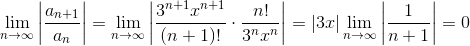Because 0 is always less than 1, the root test shows that the series converges for any value of x.

Therefore, the interval of convergence is:### Example Question #4 : Taylor's Theorem

Find the interval of convergence forof the Taylor Series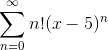.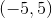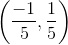Explanation:

Using the root test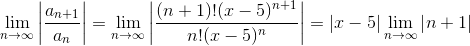and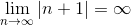. T

herefore, the series only converges when it is equal to zero.

This occurs when x=5.